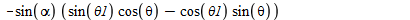## 380 Reputation

10 years, 243 days

## Pulling Numeric Values under a Root...

Maple 2015

Say I have an expression

ex:=sqrt(x)/2h

what do I tell maple to pull the 2h into the sqrt

sqrt(x/4h^2)

## keep contravariant tensor (Physics)...

restart;

with(VectorCalculus);

x := Vector([VectorCalculus:-`*`(VectorCalculus:-`+`(R, VectorCalculus:-`*`(r, cos(p))), cos(t)), VectorCalculus:-`*`(VectorCalculus:-`+`(R, VectorCalculus:-`*`(r, cos(p))), sin(t)), VectorCalculus:-`*`(r, sin(p))]);

s := [p, t];

g := Matrix(nops(s), nops(s));

for i to nops(s) do

for j to nops(s) do

g[i, j] := simplify(DotProduct(diff(x, s[i]), diff(x, s[j])))

end do

end do;

g;

with(Physics);

Setup(dimension = 2);

Coordinates(X = s);

Setup(metric = g);

g_[];

g_[mu, nu].Ricci[mu, nu]

I have one question already here: In the last step I calculate the Riemann-Scalar.

I was wondering if also this works:

SubstituteTensorIndices({rho = mu, sigma = nu}, Riemann[mu, nu, rho, sigma, Array])

but it is kinda weird. The LHS is the expression I want and the RHS keeps this Array format. What do I need to specify instead of Array in order for this to work properly...

Original Question:

Further I do the following...

Define(A[mu], F);

F[mu, nu] := D_[mu](A[nu](X));

g_[mu, nu].F[`~mu`, `~nu`];

SumOverRepeatedIndices(%);

SubstituteTensorIndices(nu = `~mu`, F[mu, nu]);

SumOverRepeatedIndices(%)

The first result using g_[mu,nu] gives me a contravariant D_ and a covariant A while the second approach (SubstituteTensorIndices) gives me the same result with D_ being covariant and A now being contravariant.

I tried to enforce the contravariance with `~mu` but it didn't work.

So is there a way to enforce this?

A last question:

If I replace D_ above by d_ the partial derivatives do not expand out.

What is the reason for this?

Similarly

Christoffel[mu, nu, nu].A[mu];

SumOverRepeatedIndices(%)

does not give me the real expression in terms of each component A and A the first time I use SumOverRepeatedIndices...I need to do it twice, why is that?

I'm also happy about general advices to improve the way I implemented everything.

Like is there any meaning for signature() if I specify the metric as I did above?

What is better to use here: Physics or Tensor Package?

## using @ for multiple applies...

Maple 17

Say I want to use multiples commands on an expression

simplify(expand(eval(expr,x=y+1)))

Now similar I could use

(simplify@expand)(expr)

but how do I do it with sth like eval which needs extra arguments

(simplify@expand@eval(x->y, x=y+1))(f)

??

## combine while excluding a variable...

Maple 17

I have this expression:

-sin(alpha)*(sin(theta1)*cos(theta)-cos(theta1)*sin(theta))which can be combined using a trigonmetric formula...

However when using combine() it also tried to sneak alpha into the result...

Is it possible to freeze the variable alpha when combining?

## Contraction in Physics package...

Maple 17

So I have the following:

restart;
with(Physics):
Setup(mathematicalnotation=true,coordinatesystems=cartesian);
Define(A[mu](X));

First of all X ist defined in terms of (x,y,z,t). Is is also possible to define it in terms of (t,x,y,z) with t being mu=0.

When I do

alias(X=(t,x,y,z))

this applies only to this one vector and

d_ is still the time differentiation, right?

Then I wanted to construct the divergence

d_[`~mu`] A[mu](X);
TensorArray(%,performsumoverrepeatedindices=true);

This should give me sth like d1 A1 + ... + d4 A4, but it stays with d_mu A_mu ?!?

Why?

Is there also a "function" which does the contraction?

So if I have

f[mu,nu]=d_[mu] A[nu](X)

eval(f[mu,nu],nu=mu)

contract(%)

??

﻿